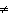Name:    mhf4u - 2.1

Multiple Choice
Identify the choice that best completes the statement or answers the question.

1.

If x3 – 4x2 + 5x – 6 is divided by x – 1, then the restriction on x is
 a. x–4 c. x1 b. x–1 d. no restrictions

2.

What is the remainder when x4 + 2x2 – 3x + 7 is divided by x + 2?
 a. 25 c. 37 b. 13 d. 9

3.

If 2x3 – 9x2 + 4x – 7 is divided by x – 3 to give a quotient of 2x2 – 3x – 5 and
a remainder of –22 , then which of the following is true?
 a. 2x3 – 9x2 + 4x – 7 = (x – 3)(2x2 – 3x – 5) + 22 b. 2x3 – 9x2 + 4x – 7 = (x – 3)(2x2 – 3x – 5) – 22 c. (x – 3)(2x2 – 3x – 5) = 22 d. (x – 3)(2x2 – 3x – 5) = –22